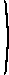# Mathematical methods for economic theory

Martin J. Osborne

A quadratic form in many variables is the sum of several terms, each of which is a constant times the product of exactly two variables.
Definition
A quadratic form in n variables is a function Q for which
 Q(x1, ..., xn) = b11x21 + b12x1x2 + ... + bijxixj + ... + bnnx2n = ∑ni=1 ∑nj=1 bijxixj
for all (x1, ..., xn), where bij for i = 1, ..., n and j = 1, ..., n are constants.

Example
The function
 Q(x1, x2) = 2x21 + 4x1x2 − 6x2x1 − 3x22
is a quadratic form in two variables.

We can write the quadratic form in this example as

Q(x1x2)  =  (x1x22 4−6 −3
·x1x2
.
Because 4x1x2 − 6x2x1 = −2x1x2, we can alternatively write it as
Q(x1x2)  =  (x1x22 −1−1 −3
·x1x2
.
In this way of writing the quadratic form, the matrix is symmetric.

We can in fact write any quadratic form as

Q(x) = x'Ax
where x is the column vector of xi's and A is a symmetric n × n matrix for which the (ij)th element is aij = (1/2)(bij + bji). The reason is that xixj = xjxi for any i and j, so that
 bijxixj + bjixjxi = (bij + bji)xixj = (1/2)(bij + bji)xixj + (1/2)(bji + bij)xjxi.

Example
Let Q(x1x2x3) = 3x2
1
+ 3x1x2 − x2x1 + 3x1x3 + x3x1 + 2x2x3 + 4x3x2 − x2
2
+ 2x2
3
. That is, a11 = 3, a12 = 3, a21 = −1, etc. We have Q(x) = x'Ax where
A =3 1 21 −1 3 2 3 2
.

Subsequently, when representing a quadratic form as x'Ax we always take the matrix A to be symmetric.